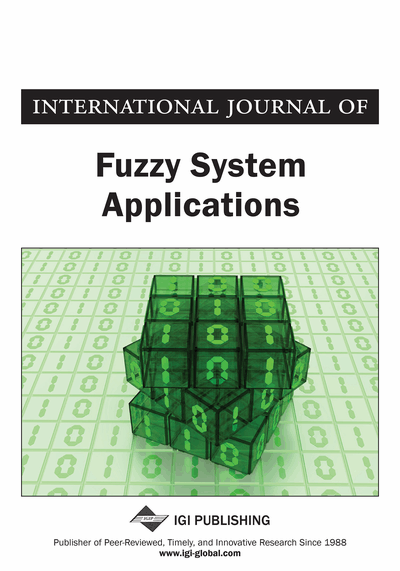# A Valid Scheme to Evaluate Fuzzy Definite Integrals by Applying the CADNA Library

DOI: 10.4018/IJFSA.2017100101
OnDemand:
(Individual Articles)
Available
\$37.50
No Current Special Offers

## Abstract

The aim of this paper is to estimate the value of a fuzzy integral and to find the optimal step size and nodes via the stochastic arithmetic. For this purpose, the fuzzy Romberg integration rule is considered as an integration rule, then the CESTAC (Controle et Estimation Stochastique des Arrondis de Calculs) method is applied which is a method to describe the discrete stochastic arithmetic. Also, in order to implement this method, the CADNA (Control of Accuracy and Debugging for Numerical Applications) is applied which is a library to perform the CESTAC method automatically. A theorem is proved to show the accuracy of the results by means of the concept of common significant digits. Then, an algorithm is given to perform the proposed idea on sample fuzzy integrals by computing the Hausdorff distance between two fuzzy sequential results which is considered to be an informatical zero in the termination criterion. Three sample fuzzy integrals are evaluated based on the proposed algorithm to find the optimal number of points and validate the results.
Article Preview
Top

## 1. Introduction

The applications of fuzzy mathematics and fuzzy logic play major rule in various areas of mathematics, physics and engineering (Wei et al., 2016; Wei et al., 2016; Kumar et al.; 2016). One of the important topics in fuzzy mathematics is to evaluate fuzzy integrals which was investigated by many authors such as (Goetschel & Voxman, 1986; Kaleva, 1987; Nanda, 1989; Ralescu & Adams, 1980; Wang, 1984; Zimmerman, 1987; Wu, 2000; Bede & Gal, 2005; Fariborzi Araghi & Khadem, 2011; Pasrija 2013; Fariborzi Araghi & Khadem, 2014). Also, Allahviranloo (2005a) used the Romberg integration rule (Atkinson, 1993; Dutka, 1984; Evans & Megson, 1986; Havie, 1986; Iyengar & Jain, 2009; Stoer & Bulirsch, 2002; Tseng & Lee, 2008) in fuzzy case as an accurate and efficient scheme to approximate the fuzzy integrals.

In these works, a fixed number of points and step size have been considered to apply the given numerical integration rule. Also, the exact solution of all examples must be accessible to compare the approximate and the exact solutions. The packages like Matlab, Mathematica or Maple were applied to implement the given algorithm which are worked based on the floating-point arithmetic. In this case, the accuracy of results cannot be estimated and the results are not optimized. Also, for the problems which the exact solution is not accessible the given algorithms cannot be applied, since the distance between the exact and approximate solutions is considered as the obtained error. Furthermore, by changing the step size, the results are changed. So, the best approximation in computer point of view cannot be computed and the optimal number of points cannot be found. This means the results may not valid informatically.

The CESTAC method is based on a probabilistic approach of the round-off error propagation which replaces the floating-point arithmetic by the stochastic arithmetic which was developed by Vignes and Laporte (Vignes; 1993). Also, the CADNA library, which was designated by Chesneaux (Chesneaux,1998; Chesneaux & Vignes, 1992; Jezequel & Chesneaux, 2004; Vignes, 1993) in order to automatic implementation of the CESTAC method was applied to validate the results of different problems such as (Chesneaux, 1990; Chesneaux, 1992; Chesneaux & Vignes, 1992; Chesneaux, 1994; Toutounian,1997; Chesneaux,1998; Chesneaux & Jezequel, 1998; Khojasteh Salkuyeh & Toutounian, 2009; Khojasteh Salkuyeh & Toutounian, 2006; Abbasbandy & Fariborzi Araghi, 2002a; Abbasbandy & Fariborzi Araghi, 2002b; Abbasbandy & Fariborzi Araghi, 2002c; Jezequel & Chesneaux, 2004; Abbasbandy & Fariborzi Araghi, 2004; Khojasteh Salkuyeh & Toutounian, 2006; Khojasteh Salkuyeh & Toutounian, 2009; Fariborzi Araghi & Noeighdam, 2016).

## Complete Article List

Search this Journal:
Reset
Volume 12: 1 Issue (2023)
Volume 11: 4 Issues (2022)
Volume 10: 4 Issues (2021)
Volume 9: 4 Issues (2020)
Volume 8: 4 Issues (2019)
Volume 7: 4 Issues (2018)
Volume 6: 4 Issues (2017)
Volume 5: 4 Issues (2016)
Volume 4: 4 Issues (2015)
Volume 3: 4 Issues (2013)
Volume 2: 4 Issues (2012)
Volume 1: 4 Issues (2011)
View Complete Journal Contents Listing